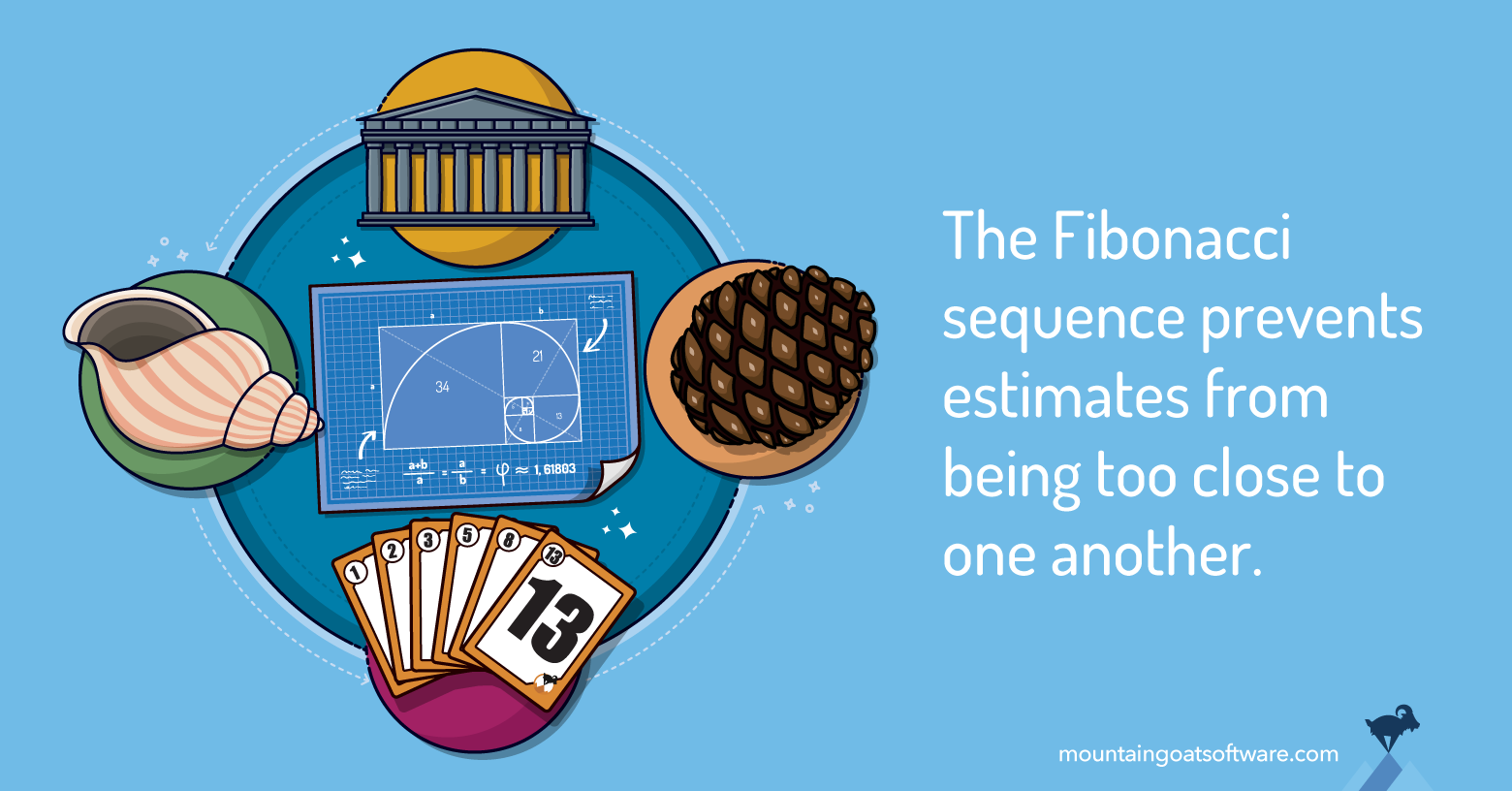# Why the Fibonacci Sequence Works Well for Estimating

If you’ve ever done agile estimating with story points or used Planning Poker®, you may have used either the Fibonacci sequence or a modified Fibonacci sequence.

The traditional Fibonacci series is 1, 2, 3, 5, 8, 13, 21, 34, 55 and so on. Each number is the sum of the two preceding numbers.

Years ago I began having teams estimate with a modified Fibonacci series: 1, 2, 3, 5, 8, 13, 20, 40 and 100.

I'll get into why I modified the larger numbers a bit later. But let's start with why I recommend the Fibonacci series as story point values instead of a sequential series (1, 2, 3, 4, 5) or even numbers (2, 4, 6, 8, 10).

It’s because numbers that are too close to one another are impossible to distinguish as estimates.

## Weber’s Law & Agile Estimation

Imagine being handed two weights—one is one kilogram (2.2 pounds) and the other is two kilograms (4.4 pounds). With one in each hand but not able to see which is which, you can probably distinguish them. The two kg weight will feel noticeably heavier.

Imagine instead being handed a 20kg weight and a 21kg weight. They are the same one kg difference as the one and two kg weights. But you would have a much harder time identifying the heavier of the two weights.

This is due to Weber’s Law. Weber’s Law states that the difference we can identify between objects is given by a percentage.

The difference from one to two kilograms is 100%. You can probably distinguish the weight of items that differ by 100%.

The difference between 20 and 21kg, however, is only 5%. You probably can’t tell the difference. (I know I can’t.) And if you could, it would mean you should be able to distinguish between a 1.00 kg weight and a 1.05 kg weight, as that would also be 5%.

The values in the Fibonacci scale work well because they roughly correspond to Weber’s Law. After the two (which is 100% bigger than one), each number is about 60% larger than the preceding value.

According to Weber’s Law, if we can distinguish a 60% difference in effort between two estimates, we can distinguish that same percentage difference between other estimates.

So, the Fibonacci values work well because they increase by about the same proportion each time.

## Modifying the Fibonacci Sequence

Early agile teams I worked with made use of this and estimated with the real Fibonacci sequence.

Ultimately, though, we learned that an estimate of 21 story points implied a precision we couldn’t support. Stakeholders would look at the 21 and be impressed that we called it 21 rather than rounding it to 20 or even 25.

This led us to start using 20 rather than 21. Once we’d deviated from the Fibonacci sequence once, we felt free to do so further.

That led to experimentation in which we introduced 40 and 100. Those worked well because they represented 100% and 150% increases over the preceding numbers. This was much larger than the 62% of the Fibonacci sequence.

This wasn’t a violation of Weber’s Law because Weber’s Law has been shown not to hold at extreme values. Estimates such as 40 and 100 could, perhaps, be thought of as extreme values.

## Experimenting with Planning Poker Sequences

Up until 2007, teams I worked with experimented with both the modified Fibonacci sequence and a simple doubling of numbers—1, 2, 4, 8, 16, 32.

Each worked equally well. Most teams had a strong preference for one or the other, but I could find no clear evidence that either sequence was better than the other.

But in 2007, we began printing Planning Poker Cards, which we sold at cost, distributed at various agile events, and that I use in some in-person courses.

To keep printing costs down, I had to choose between these two sequences. At the time, I just slightly favored the modified Fibonacci sequence. And so I made the call to go with that.

Once the modified Fibonacci series began to appear on thousands of decks a year, the sequence gained popularity at the expense of simply doubling the values. My slight preference for 1, 2, 3, 5, 8, 13... over 1, 2, 4, 8, 16... came from being in a lot of meetings with the latter sequence in which discussions were always “Is this product backlog item double the size of the other one?”

All discussions about user stories were about whether something was double, quadruple, etc. That concerned me slightly because I didn’t see it with teams using the modified Fibonacci sequence. These team members discussions were more about the work involved in the user stories rather than “Is this one 60% more effort?” Those seemed like healthier discussions, even though I had no way of measuring that.

These days I remain fairly impartial between the two sets of values. Either set of values exhibits attributes of Weber’s Law.

## What Do You Think?

What’s your experience with different estimating sequences? Which values do you use and do they work for your team? Please share your thoughts in the comments section below.

Posted:

## Tagged: